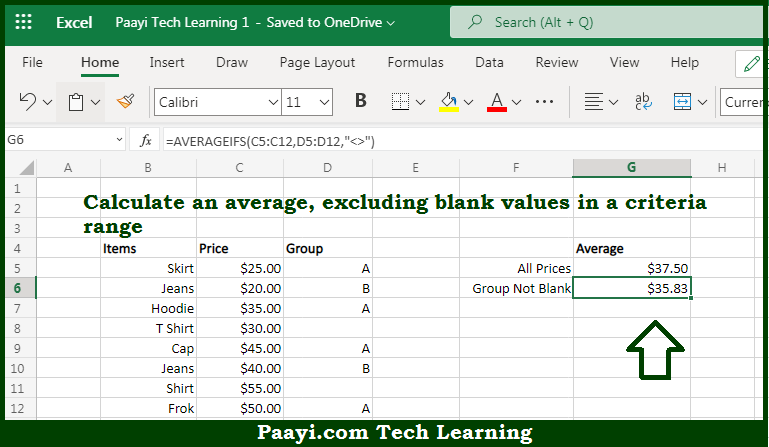# Learn How to AVERAGE If Criteria Not Blank in Microsoft Excel

Written by | 0 Comments | 612 Views

In this article, you will learn how to SUM various things in Microsoft Excel using a single/combination(s) of functions. You will also know how to AVERAGE If Criteria Not Blank and see the generic formula.

AVERAGE If Criteria Not Blank in Microsoft Excel

The main purpose of this formula is to calculate an average, excluding blank values in a criteria range. Here we will learn how to average if the criteria are not blank in the workbook in Microsoft Excel. That implies, with the help of a formula based on the AVERAGEIFS function you can able to calculate an average, excluding blank values in a criteria range. So, with the help of this formula, you can able to average if the criteria are not blank in the workbook in Microsoft Excel.

General Formula to AVERAGE If Criteria Not Blank

=AVERAGEIFS(range1,range2,"<>")

The Explanation for the AVERAGE If Criteria Not BlankSo we know that with the help of the given formula above you can able to calculate an average, excluding blank values in a criteria range. Here we will learn how to average if the criteria are not blank in the workbook in Microsoft Excel. As we know that the AVERAGEIFS function is used to average ranges based on multiple criteria. So, with the help of this formula, you can able to average if the criteria are not blank in the workbook in Microsoft Excel.